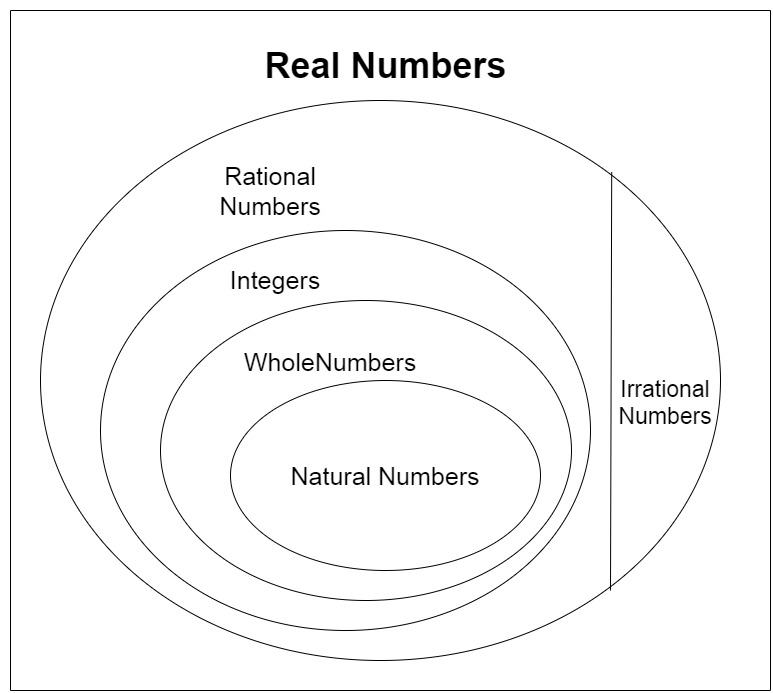Open in App
Not now

# Class 9 NCERT Solutions- Chapter 1 Number System – Exercise 1.1

• Last Updated : 17 Nov, 2020

### Question 1: Is zero a rational number? Can you write it in the form p/q, where p and q are integers and q ≠ 0?

Solution:

A number is a rational number if it can be written in the form of p/q, where p and q are integers and q ≠ 0

• Therefore, we can write 0 in the form of 0/1, 0/2, 0/3, 0/4.
• As well as, q can be a negative integer also, 0/-1, 0/-2, 0/-3, 0/-4.
So we can see 0 can be written in p/q, form hence 0 is a rational number.

### Question 2: Find six rational numbers between 3 and 4.

Solution:

We can find infinite rational numbers between 3 and 4.
Now we have to find 6 rational numbers between 3 and 4 we will multiply and divide both the numbers 3 and 4 by (6 + 1) 7.

• 3 = 3 × 7/7 = 21/7
• 4 = 4 × 7/7 = 28/7

Hence the 6 rational numbers are 23/7, 24/7, 25/7, 26/7, 27/7, and 28/7.

### Question 3: Find five rational numbers between 3/5 and 4/5.

Solution:

We need to find 5 rational numbers between 3/5 and 4/5.
Multiply both numerator and denominator by (5 + 1) 6.

•  3/5 = 3/5 × 6/6 = 18/30
•  4/5 = 4/5 × 6/6 = 24/30

Hence, the 5 rational number between 3/5 and 4/5 are 19/30, 20/30, 21/30, 22/30 and 23/30.

### (iii) Every rational number is a whole number.

Solution:

(i) Every natural number is a whole number.

True

Explanation: The natural numbers starts from 1, 2, 3, 4 …..
The whole number starts from 0, 1, 2, 3 , 4 …..
Here it is clearly seen that whole number contains all the natural numbers and 0 also
Therefore, every natural number is a whole number but not every whole number is not a natural number as 0 is a whole number but not a natural number.

(ii) Every integer is a whole number.

False

Explanation: Integers are the numbers that have both positive and negative numbers including 0,

Example: …..-4, -3, -2, -1, 0, 1, 2, 3, 4 ……
Whereas whole numbers begin from 0 to infinite
Example: 0, 1, 2, 3, 4…..
Here we can see every whole number is an integer but not all the integers are the whole number.

(iii) Every rational number is a whole number.

False

Explanation: Rational numbers are the numbers that can be written in the form of p/q where q ≠ 0.

Example: 0, 2/5, 4/17, 7/15 …..

Whole numbers are that starts from 0 to infinity
As we know whole numbers can be written in the form of 0/1, 1/1, 2/1, …

Thus, every whole number is a rational number but every rational number is not a whole number.My Personal Notes arrow_drop_up